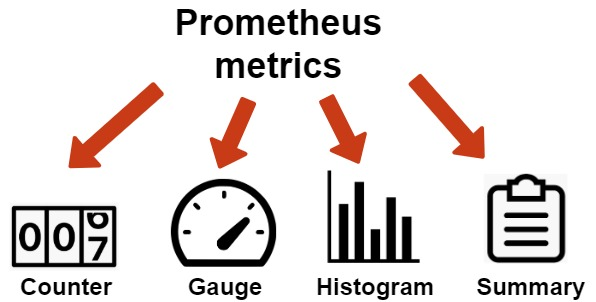# Prometheus 常用 PromQL 语句

soft_007

1收藏

PromQL 是 Prometheus 提供的一个函数式的表达式语言，可以使用户实时地查找和聚合时间序列数据。表达式计算结果可以在图表中展示，也可以在 Prometheus表达式浏览器中以表格形式展示，或者作为数据源，以 HTTP API 的方式提供给外部系统使用。PromQL 虽然以 QL 结尾，但是它不是类似 SQL 的语言，因为在时间序列上执行计算类型时，SQL 语言相对缺乏表达能力。而 PromQL 语言表达能力非常丰富，可以使用标签进行任意聚合，还可以使用标签将不同的标签连接到一起进行算术运算操作。内置了时间和数学等很多函数可以使用。``my_metric_name``

``my_metric_name[5m]``

``my_metric_name{label1="value1", label2="value2"}``

``my_metric_name{label1!="value1", label2=~"regex1", label3!~"regex2"}``

• =: 等于
• !=: 不等于
• =~: 正则匹配
• !~: 正则不匹配

Counter 的增长速率

``rate(http_requests_total[5m])``

1分钟时间窗口中最后两个样本计算的每秒增长速率：

``irate(http_requests_total[1m]``

``increase(http_requests_total[1h])``

``sum(my_metric_name)``

``sum by(method, path) (my_metric_name)``

without 用于从计算结果中移除列举的标签，而保留其它标签，by 则正好相反，结果向量中只保留列出的标签，其余标签则移除：

``sum without(method, path) (my_metric_name)``

``left_metric + right_metric``

``left_metric + on(instance, job) right_metric``

``left_metric + ignoring(instance, job) right_metric``

``left_metric + on(instance, job) group_left right_metric``

``left_metric + on(instance, job) group_left(version) right_metric``

``left_metric or right_metric``

``left_metric and right_metric``

``left_metric unless right_metric``

``left_metric and on(job, instance) right_metric``

``left_metric > 1024``

``left_metric > right_metric``

``left_metric > bool right_metric``

``left_metric > on(job, instance) right_metric``

``histogram_quantile(0.9, rate(my_request_durations_seconds_bucket[5m]))``

``histogram_quantile( 0.9, sum by(le, path, method) ( rate(my_request_durations_seconds_bucket[5m]) ))``

Gauges 的变化

``delta(my_gauge[1h])``

``predict_linear(my_gauge[4h], 3600)``

``time()``

``time() - my_batch_job_last_success_timestamp_seconds``

``time() - my_batch_job_last_success_timestamp_seconds > 3600``

``absent(my_metric_name)``

``absent_over_time(my_metric_name[5m])``

``label_join(my_metric_name, "-", "label1", "label2")``

``label_replace(my_metric, "dest-label", "\$1", "source-label", "(.*):.*")``

• 基础：https://prometheus.io/docs/prometheus/latest/querying/basics/
• 操作符：https://prometheus.io/docs/prometheus/latest/querying/operators/
• 函数：https://prometheus.io/docs/prometheus/latest/querying/functions/
• 示例：https://prometheus.io/docs/prometheus/latest/querying/examples/

1帖子
视频
声望
粉丝
最近发布
相关问题
社区精华内容